### CONTRAST Statement

CONTRAST ’label’ row-description <,, row-description> </ options> ;

where a row-description is defined as follows:

<@n> effect values <…<@n> effect values >

The CONTRAST statement constructs and tests linear functions of the parameters in the MODEL statement or effects listed in the LOGLIN statement. Each set of effects (separated by commas) specifies one row or set of rows of the matrix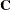that PROC CATMOD uses to test the hypothesis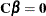.

CONTRAST statements must be preceded by the MODEL statement, and by the LOGLIN statement, if one is used. You can specify the following terms in the CONTRAST statement.

label

specifies up to 256 characters of identifying information displayed with the test. The ’label’ is required.

effect

is one of the effects specified in the MODEL or LOGLIN statement, INTERCEPT (for the intercept parameter), or ALL_PARMS (for the complete set of parameters).

The ALL_PARMS option is regarded as an effect with the same number of parameters as the number of columns in the design matrix. This is particularly useful when the design matrix is input directly, as in the following example:

model y=(1 0 0 0,
1 0 1 0,
1 1 0 0,
1 1 1 1);
contrast 'Main Effect of B' all_parms 0 1 0 0;
contrast 'Main Effect of C' all_parms 0 0 1 0;
contrast 'B*C Interaction ' all_parms 0 0 0 1;

values

are numbers that form the coefficients of the parameters associated with the given effect. If there are fewer values than parameters for an effect, the remaining coefficients become zero. For example, if you specify two values and the effect actually has five parameters, the final three are set to zero.

@n

points to the parameters in the nth set when the model has a separate set of parameters for each of the response functions. The @n notation is seldom needed. It enables you to test the variation among response functions in the same population. However, it is usually easier to model and test such variation by using the _RESPONSE_ effect in the MODEL statement or by using the ALL_PARMS designation. Usually, contrasts are performed with respect to all of the response functions, and this is what the CONTRAST statement does by default (in this case, do not use the @n notation).

For example, if there are three response functions per population, then the following contrast results in a three-degree-of-freedom test comparing the first two levels of A simultaneously on the three response functions.

contrast 'Level 1 vs. Level 2' A 1 -1 0;


If, however, you want to specify a contrast with respect to the parameters in the nth set only, then use a single @n in a row-description. For example, the following statement tests that the first parameter of A and the first parameter of B are zero in the third response function:

contrast 'A=0, B=0, Function 3'  @3  A 1  B 1;


To specify a contrast with respect to parameters in two or more different sets of effects, use @n with each effect. For example:

contrast 'Average over Functions' @1 A 1 0 -1
@2 A 1 1 -2;


When the model does not have a separate set of parameters for each of the response functions, the @n notation is invalid. This type of model is called AVERAGED. For details, see the description of the AVERAGED option and the section Generation of the Design Matrix.

You can specify the following options in the CONTRAST statement after a slash.

ALPHA=value

specifies the significance level of the confidence interval for each contrast when the ESTIMATE= option is specified. The default is ALPHA=0.05, resulting in a 95% confidence interval for each contrast.

ESTIMATE=keyword
EST=keyword

requests that each individual contrast (that is, each row,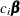, of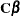) or exponentiated contrast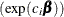be estimated and tested. PROC CATMOD displays the point estimate, its standard error, a Wald confidence interval, and a Wald chi-square test for each contrast. The significance level of the confidence interval is controlled by the ALPHA= option.

You can estimate the contrast or the exponentiated contrast, or both, by specifying one of the following keywords:

PARM

specifies that the contrast itself be estimated.

EXP

specifies that the exponentiated contrast be estimated.

BOTH

specifies that both the contrast and the exponentiated contrast be estimated.

#### Specifying Contrasts

PROC CATMOD is parameterized differently than PROC GLM, so you must be careful not to use the same contrasts that you would with PROC GLM. Since PROC CATMOD uses full-rank parameterizations, all estimable parameters are directly estimable without involving other parameters.

For example, suppose a classification variable A has four levels and uses the default parameterization (PARAM=EFFECT). Then there are four parameters (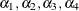), of which PROC CATMOD uses only the first three. The fourth parameter is related to the others by the equation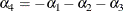To test the first versus the fourth level of A, you would test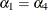, which is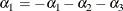or, equivalently,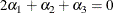Therefore, you would use the following CONTRAST statement:

contrast '1 vs. 4' A 2 1 1;


To contrast the third level with the average of the first two levels, you would test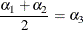or, equivalently,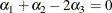Therefore, you would use the following CONTRAST statement:

contrast '1&2 vs. 3' A 1 1 -2;


Other CONTRAST statements are constructed similarly. For example:

contrast '1 vs. 2    '  A  1 -1  0;
contrast '1&2 vs. 4  '  A  3  3  2;
contrast '1&2 vs. 3&4'  A  2  2  0;
contrast 'Main Effect'  A  1  0  0,
A  0  1  0,
A  0  0  1;


The actual form of thematrix depends on the effects in the model. The remaining examples in this section assume a single response function for each population.

Recall that the statements to test the first versus the fourth level of A are as follows:

proc catmod;
model y=a;
contrast '1 vs. 4' A 2 1 1;
run;


Since the first parameter corresponds to the intercept, thematrix for the preceding statements is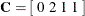But suppose you have a variable B with three levels and you use the following statements:

proc catmod;
model y=b a;
contrast '1 vs. 4' A 2 1 1;
run;


Then the CONTRAST statement produces thematrix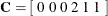since the first parameter corresponds to the intercept and the next two correspond to the B main effect.

You can also use the CONTRAST statement to test the joint effect of two or more effects in the MODEL statement. For example, the joint effect of A and B in the previous model has five degrees of freedom and is obtained by specifying the following:

contrast 'Joint Effect of A&B' A 1 0 0,
A 0 1 0,
A 0 0 1,
B 1 0,
B 0 1;


The ordering of variable levels is determined by the ORDER= option in the PROC CATMOD statement. Whenever you specify a contrast that depends on the order of the variable levels, you should verify the order from the Population Profiles table, the Response Profiles table, or the One-Way Frequencies table.